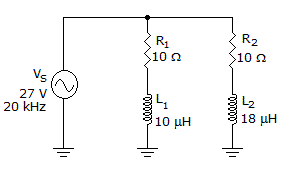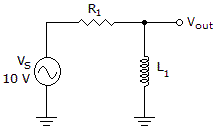# Electronics - RL Circuits

Exercise : RL Circuits - General Questions
6.Calculate the voltage dropped across L2 in the given circuit.

18 V
6 V
13.5 V
0 V
Explanation:
No answer description is available. Let's discuss.

7.
What is the magnitude of the phase angle of a 24 Vac parallel RL circuit when R = 45and XL = 1100?
0.001°
2.3°
87.6°
89.9°
Explanation:
No answer description is available. Let's discuss.

8.Which of the following statements is true if the frequency decreases in the circuit in the given circuit?

The phase angle decreases.
VR decreases.
IT decreases.
VS decreases.
Explanation:
No answer description is available. Let's discuss.

9.
What is the magnitude of the phase angle between the source voltage and current when a 100 mH inductor with an inductive reactance of 6 kand a 1 kresistor are in series with a source?
0.1°
9.0°
61.0°
81.0°
Explanation:
No answer description is available. Let's discuss.

10.Which of the following statements is true if R1 opens in the circuit in the given circuit?

IL2 increases.
ZT decreases.
VS increases.
VL1 equals 0 V.